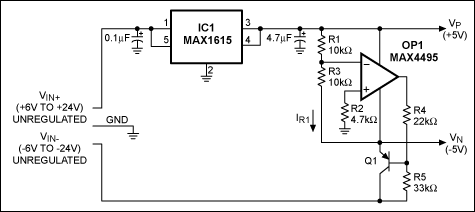# Spare Op Amp Generates Its Own Regulated Negative Supply

### 要約

This application note shows how to use a spare op amp to generate a regulated negative voltage supply, used to power the op amp itself as well as other low current circuitry. The regulated negative supply is generated from a regulated positive voltage and an unregulated negative voltage.

This design idea appeared in the April 14, 2006 issue of EE Times.

For systems that require low current (100mA or less) and include a spare op amp along with unregulated positive and negative voltages, the circuit of Figure 1 generates a regulated positive and negative supply voltage. The op amp then operates from the supply rails that it has helped to generate (±5V in this case). Figure 2 shows the power-up response. The circuit achieves regulation regardless of which unregulated voltage is applied first.Figure 1. This circuit derives a regulated ± voltage from ± unregulated input voltages.Figure 2. The power-up response for the Figure 1 circuit.
CH1 = regulated -5V output
CH2 = negative supply voltage
CH3 = regulated +5V output
CH4 = positive supply voltage

By controlling the base drive to Q1, the op amp maintains equilibrium at its inverting input, and thereby ensures that VN = -VP(R3/R1). Resistor R5 provides the initial base current to Q1 until OP1 takes over. When that happens, OP1, Q1, and R1, R3, R4, and R5 form a negative feedback network that regulates VN to -VP(R3/R1):

VOP1+ = VOP1-
VOP1+ = 0V
Using Superposition:
VOP1-' = VP(R3/(R3+R1)
VOP1-'' = (VN(R1/(R1+R3)
VOP1- = VOP1-' + VOP1-''
VOP1- = VP(R3/(R3+R1)) + VN(R1/(R1+R3))
VN = -VP(R3/(R3+R1))((R1+R3)/R1)
Therefore:
VN = -VP(R3/R1)
Alternatively:
IR1 = VP/R1
Therefore:
VN = -IR1 × R3 = (-VP/R1)R3

The allowable range of the unregulated negative supply voltage is -6V to -24V. For a wider input-supply range, either replace Q1 with a higher gain transistor or lower the resistance of R4 and R5. VN can deliver up to 100mA without distortion, but VP is limited by the reference of IC1 to a maximum of 30mA, with a VP reduction at that level of ~0.3V. (For higher positive load currents you can substitute a different positive regulator.)

IBIAS is negligible for the op amp shown. To minimize the IBIAS error for other op amps, R2 should have the value R1||R3.